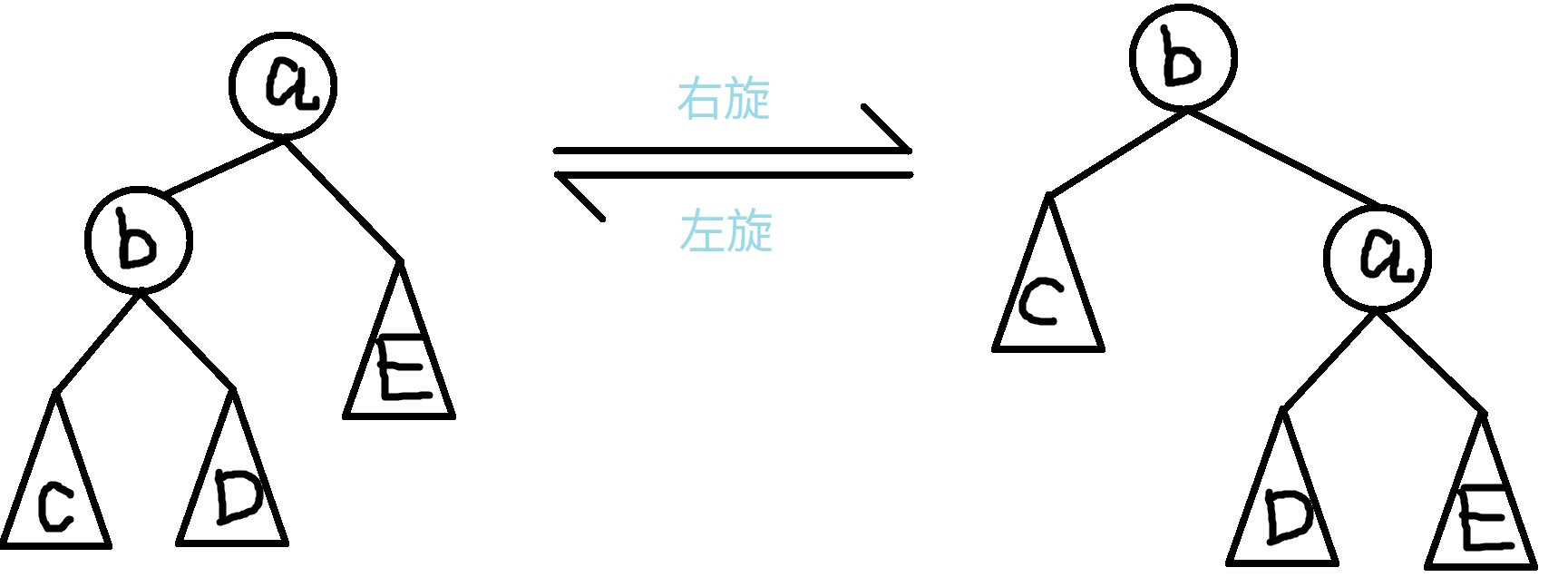# Treap 笔记

Treap = Tree + Heap

## 二叉搜索树（BST）

• 设 $x$ 是二叉搜索树中的一个结点。如果 $y$ 是 $x$ 左子树中的一个结点，那么 $y.key \lt x.key$。如果 $y$ 是 $x$ 右子树中的一个结点，那么 $y.key \gt x.key$。

• BST 上的基本操作所花费的时间与这棵树的高度成正比。对于一个有 $n$ 个结点的二叉搜索树中，这些操作的最优时间复杂度为 $O(\log n)$，最坏为 $O(n)$。随机构造这样一棵二叉搜索树的期望高度为 $O(\log n)$。然而，当这棵树退化成链时，则同样的操作就要花费 $O(n)$ 的最坏运行时间。

## Treap

### 旋转式 Treap

#### 旋转#### 删除

1. 没有子结点：直接就成空的了
2. 只有一个子结点：把被删除结点设成它仅有的儿子即可
3. 有两个子结点：选出两个儿子中 $fix$ 值较大的一个，通过旋转操作把它设成新的根，这样要删除的结点就只有一个儿子了，按照情况 2 处理。这种方法保证满足了 BST 和最大堆的性质。

P3369 【模板】普通平衡树 - 洛谷 为例，代码如下。

  1 2 3 4 5 6 7 8 9 10 11 12 13 14 15 16 17 18 19 20 21 22 23 24 25 26 27 28 29 30 31 32 33 34 35 36 37 38 39 40 41 42 43 44 45 46 47 48 49 50 51 52 53 54 55 56 57 58 59 60 61 62 63 64 65 66 67 68 69 70 71 72 73 74 75 76 77 78 79 80 81 82 83 84 85 86 87 88 89 90 91 92 93 94 95 96 97 98 99 100 101 102 103 104 105 106 107 108 109 110 111 112 113 114 115 116 117 118 119 120 121 122 123 124 125 126 127 128 129 130 131 132 133 134 135 136 137 138 139 140 141 142 143 144 145 146 147 148 149 150 151 152 153 154 155 156 157  #include #include #include #include #include #include using namespace std; const int INF = 1000000009; struct NODE { int val, fix, size; // size 指树的总结点数 NODE *left, *right; NODE(const int val) : val(val) { fix = rand(); left = right = NULL; size = 1; } }; void maintain(NODE *&p) { p->size = 1; if (p->left != NULL) p->size += p->left->size; if (p->right != NULL) p->size += p->right->size; } void rightRotate(NODE *&p) { NODE *tmp = p->left; p->left = tmp->right; tmp->right = p; p = tmp; maintain(tmp->right); maintain(tmp); } void leftRotate(NODE *&p) { NODE *tmp = p->right; p->right = tmp->left; tmp->left = p; p = tmp; maintain(tmp->left); maintain(tmp); } void insert(NODE *&p, const int value) { if (p == NULL) { p = new NODE(value); } else if (value <= p->val) { insert(p->left, value); if (p->left->fix < p->fix) rightRotate(p); } else { insert(p->right, value); if (p->right->fix < p->fix) leftRotate(p); } maintain(p); } int count(const NODE *p, const int value) { if (!p) return 0; if (p->val == value) return 1; if (value <= p->val) return count(p->left, value); return count(p->right, value); } void remove(NODE *&p, const int value) { if (!p) return; if (p->val == value) { if (p->left == NULL || p->right == NULL) { NODE *tmp = p; if (p->right) p = p->right; else p = p->left; delete tmp; } else if (p->left->fix < p->right->fix) { rightRotate(p); remove(p->right, value); maintain(p); } else { leftRotate(p); remove(p->left, value); maintain(p); } } else if (value < p->val) { remove(p->left, value); maintain(p); } else { remove(p->right, value); maintain(p); } } int getrank(const NODE *p, int value) { if (!p) return INF; int leftsize = 0; if (p->left != NULL) leftsize = p->left->size; if (p->val == value) return min(leftsize + 1, getrank(p->left, value)); else if (value < p->val) return getrank(p->left, value); else if (value > p->val) return leftsize + 1 + getrank(p->right, value); } int find(const NODE *p, int rank) { if (!p) return 0; int leftsize = 0; if (p->left != NULL) leftsize = p->left->size; if (leftsize >= rank) return find(p->left, rank); else if (leftsize + 1 == rank) return p->val; else return find(p->right, rank - leftsize - 1); } int getpre(const NODE *p, int value) { // 前驱 if (!p) return -INF; if (p->val >= value) return getpre(p->left, value); else return max(p->val, getpre(p->right, value)); } int getnext(const NODE *p, int value) { // 后继 if (!p) return INF; if (p->val <= value) return getnext(p->right, value); else return min(p->val, getnext(p->left, value)); } int n; NODE *root; int main() { scanf("%d", &n); while (n-- > 0) { int opt, x; scanf("%d %d", &opt, &x); if (opt == 1) { insert(root, x); } else if (opt == 2) { remove(root, x); } else if (opt == 3) { printf("%d\n", getrank(root, x)); } else if (opt == 4) { printf("%d\n", find(root, x)); } else if (opt == 5) { printf("%d\n", getpre(root, x)); } else if (opt == 6) { printf("%d\n", getnext(root, x)); } } return 0; } 

### 无旋 Treap

#### 分裂（Split）1 2 3 4 5 6 7 8 9 10 11 12 13 14 15  pair split(NODE *u, const int value) { if (u == nullptr) return make_pair(nullptr, nullptr); if (u->value > value) { auto tmp = split(u->ch, value); u->ch = tmp.second; maintain(u); return make_pair(tmp.first, u); } else { auto tmp = split(u->ch, value); u->ch = tmp.first; maintain(u); return make_pair(u, tmp.second); } } 

#### 合并（Merge）1 2 3 4 5 6 7 8 9 10 11 12 13 14  NODE *merge(NODE *l, NODE *r) { if (l == nullptr) return r; if (r == nullptr) return l; if (l->fix < r->fix) { l->ch = merge(l->ch, r); maintain(l); return l; } // else r->ch = merge(l, r->ch); maintain(r); return r; } 

#### 插入1 2 3 4 5  void insert(int value) { auto tmp = split(root, value); tmp.first = merge(tmp.first, new NODE(value)); root = merge(tmp.first, tmp.second); } 

#### 删除1 2 3 4 5 6  void erase(int value) { auto tmp = split(root, value - 1); auto tmp2 = split(tmp.second, value); delete tmp2.first; root = merge(tmp.first, tmp2.second); }